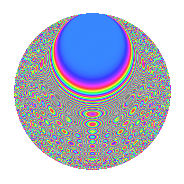# Properties

 Label 806.2.bnLevel 806 Weight 2 Character orbit bn Rep. character $$\chi_{806}(151,\cdot)$$ Character field $$\Q(\zeta_{20})$$ Dimension 320 Newforms 1 Sturm bound 224 Trace bound 0

# Related objects

## Defining parameters

 Level: $$N$$ = $$806 = 2 \cdot 13 \cdot 31$$ Weight: $$k$$ = $$2$$ Character orbit: $$[\chi]$$ = 806.bn (of order $$20$$ and degree $$8$$) Character conductor: $$\operatorname{cond}(\chi)$$ = $$403$$ Character field: $$\Q(\zeta_{20})$$ Newforms: $$1$$ Sturm bound: $$224$$ Trace bound: $$0$$

## Dimensions

The following table gives the dimensions of various subspaces of $$M_{2}(806, [\chi])$$.

Total New Old
Modular forms 928 320 608
Cusp forms 864 320 544
Eisenstein series 64 0 64

## Trace form

 $$320q + 16q^{7} + 96q^{9} + O(q^{10})$$ $$320q + 16q^{7} + 96q^{9} - 20q^{11} - 20q^{13} + 12q^{14} + 80q^{16} + 16q^{18} - 32q^{19} - 60q^{21} - 60q^{27} - 24q^{28} + 4q^{31} + 52q^{33} - 40q^{34} - 40q^{35} + 44q^{39} + 28q^{41} + 40q^{46} - 32q^{47} - 40q^{53} + 4q^{59} + 104q^{63} + 40q^{65} + 32q^{66} - 32q^{67} - 24q^{70} + 8q^{71} - 16q^{72} - 48q^{76} - 76q^{78} + 80q^{79} - 272q^{81} - 40q^{83} + 40q^{84} + 80q^{85} + 32q^{87} - 80q^{89} - 200q^{91} - 100q^{93} - 8q^{94} + 52q^{97} + 48q^{98} + O(q^{100})$$

## Decomposition of $$S_{2}^{\mathrm{new}}(806, [\chi])$$ into irreducible Hecke orbits

Label Dim. $$A$$ Field CM Traces $q$-expansion
$$a_2$$ $$a_3$$ $$a_5$$ $$a_7$$
806.2.bn.a $$320$$ $$6.436$$ None $$0$$ $$0$$ $$0$$ $$16$$

## Decomposition of $$S_{2}^{\mathrm{old}}(806, [\chi])$$ into lower level spaces

$$S_{2}^{\mathrm{old}}(806, [\chi]) \cong$$ $$S_{2}^{\mathrm{new}}(403, [\chi])$$$$^{\oplus 2}$$